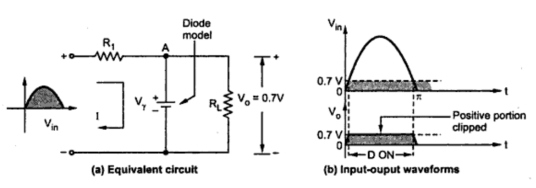### Effect of Cut-in Voltage of Diode

Parallel Clippers : Part2
Let us use linear piecewise model of diode, in which diode conducts when potential of node A becomes just equal to cut-in voltage of the diode. And once diode starts conducting, it acts as a voltage source of value 0.7 V for silicon, neglecting internal resistance rf of diode. Hence VA = 0.7 V for the conducting diode.
Note : As Vo = VA , the output voltage is 0.7 V till Vin >0.7 V.
This is shown in the Fig. 1.Fig. 1  Basic parallel clipper (Positive half cycle)

Thus almost entire positive half cycle gets clipped off.
In the negative half cycle of the input voltage Vin, when Vin is less than 0.7 V, the diode becomes reverse biased and acts as an open circuit. Hence current I flows through  R1 and RL. The output voltage is negative part of the input voltage whose magnitude is given by,
Note : Thus output follows the input and negative cycle of Vin gets reproduced at the output.
This is shown in the Fig. 2.Fig. 2  Basic parallel clipper (Negative half cycle)
The overall input-output waveforms can be shown as in the Fig. 3(a) using linear piecewise diode model.Fig. 3  Piecewise model
Transfer characteristics :
It is known that the transfer characteristics is the graph of output voltage against input voltage with time t as an implicate variable.
For the basic parallel clipper circuit we can write,
Using the equations the transfer characteristics can be obtained as shown in the Fig. 3.Fig. 4  Transfer characteristics

Note : The portion for which Vin < Vγ is called the transmitting region while the portion for which Vin > Vγ is called limiting region or clipping region.